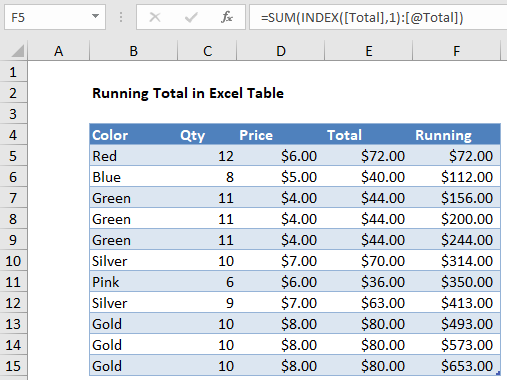## Excel Office

Excel How Tos, Tutorials, Tips & Tricks, Shortcuts

# How to create running total in an Excel Table

This tutorial shows illustrates a Running total in Excel Table.

To create a running total in an Excel Table, you can use the INDEX function set up with a structured reference.

## Formula

`=SUM(INDEX([column],1):[@column])`## Explanation

In the example shown, the formula in F5 is:

`=SUM(INDEX([Total],1):[@Total])`

When copied down the column, this formula will return a running total at each row.

### How this formula works

At the core, this formula has a simple pattern like this:

`=SUM(first:current)`

Where “first” is the first cell in the Total column, and “current” is a reference to a cell in the current row of the Total column.

Worked Example:   How to generate random number weighted probability in Excel

To get the a reference to the first cell, we use INDEX like this:

`INDEX([Total],1)`

Here, the array is the entire “Total” column and row number is 1. This works because, the INDEX function returns a reference to the first cell, not the actual value.

Worked Example:   Basic INDEX MATCH approximate in Excel

To get a reference to the current row, we use:

`[@Total]`

This is the standard structured reference syntax for “this row”.

The SUM function sums the values in the range at each row, creating a running total.

As the formula is copied down the column, the reference to the first cell doesn’t change, but the referent to the current cell changes at each row. The result is a reference that expands.

Worked Example:   How To Use AGGREGATE function to sum a range with errors in Excel

### Simple expanding range

Why not use a simple expanding range like this?

`=SUM(\$E\$5:E5)`

For some reason, this kind of mixed reference becomes corrupted in an Excel Table as rows are added. Using INDEX with a structured reference solves the problem.